﻿ C : Delete a node from the middle of Singly Linked List

# C Exercises: Delete a node from the middle of Singly Linked List

## C Singly Linked List : Exercise-8 with Solution

Write a program in C to delete a node from the middle of a Singly Linked List.

Pictorial Presentation: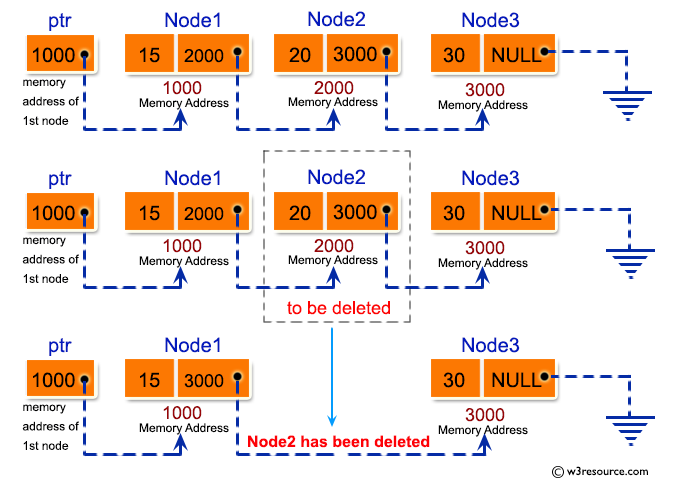Sample Solution:

C Code:

``````#include <stdio.h>
#include <stdlib.h>

struct node
{
int num;                    //Data of the node
struct node *nextptr;       //Address of the node
}*stnode;

void createNodeList(int n);     	//function to create the list
void MiddleNodeDeletion(int pos);	//function to delete a node from middle
void displayList();             	//function to display the list

int main()
{
int n,num,pos;
printf("\n\n Linked List : Delete a node from the middle of Singly Linked List. :\n");
printf("-------------------------------------------------------------------------\n");

printf(" Input the number of nodes : ");
scanf("%d", &n);
createNodeList(n);

printf("\n Data entered in the list are : \n");
displayList();

printf("\n Input the position of node to delete : ");
scanf("%d", &pos);

if(pos<=1 || pos>=n)
{
printf("\n Deletion can not be possible from that position.\n ");
}
if(pos>1 && pos<n)
{
printf("\n Deletion completed successfully.\n ");
MiddleNodeDeletion(pos);
}

printf("\n The new list are  : \n");
displayList();
return 0;
}
void createNodeList(int n)
{
struct node *fnNode, *tmp;
int num, i;

stnode = (struct node *)malloc(sizeof(struct node));
if(stnode == NULL) 			//check whether the stnode is NULL and if so no memory allocation
{
printf(" Memory can not be allocated.");
}
else
{
// reads data for the node through keyboard
printf(" Input data for node 1 : ");
scanf("%d", &num);

stnode-> num = num;
tmp = stnode;

for(i=2; i<=n; i++)
{
fnNode = (struct node *)malloc(sizeof(struct node));

if(fnNode == NULL) 		//check whether the fnnode is NULL and if so no memory allocation
{
printf(" Memory can not be allocated.");
break;
}
else
{
printf(" Input data for node %d : ", i);
scanf(" %d", &num);

fnNode->num = num;      // links the num field of fnNode with num
fnNode->nextptr = NULL; // links the address field of fnNode with NULL

tmp->nextptr = fnNode; // links previous node i.e. tmp to the fnNode
tmp = tmp->nextptr;
}
}
}
}

void MiddleNodeDeletion(int pos)
{
int i;
struct node *toDelMid, *preNode;

if(stnode == NULL)
{
printf(" There are no nodes in the List.");
}
else
{
toDelMid = stnode;
preNode = stnode;

for(i=2; i<=pos; i++)
{
preNode = toDelMid;
toDelMid = toDelMid->nextptr;

if(toDelMid == NULL)
break;
}
if(toDelMid != NULL)
{
if(toDelMid == stnode)
stnode = stnode->nextptr;

preNode->nextptr = toDelMid->nextptr;
toDelMid->nextptr = NULL;
free(toDelMid);
}
else
{
printf(" Deletion can not be possible from that position.");
}
}
}

void displayList()
{
struct node *tmp;
if(stnode == NULL)
{
printf(" No data found in the list.");
}
else
{
tmp = stnode;
while(tmp != NULL)
{
printf(" Data = %d\n", tmp->num);   // prints the data of current node
tmp = tmp->nextptr;                 // advances the position of current node
}
}
}
```
```

Sample Output:

```Linked List : delete a node from the middle of Singly Linked List. :
-------------------------------------------------------------------------
Input the number of nodes : 3
Input data for node 1 : 2
Input data for node 2 : 5
Input data for node 3 : 8

Data entered in the list are :
Data = 2
Data = 5
Data = 8

Input the position of node to delete : 2

Deletion completed successfully.

The new list are  :
Data = 2
Data = 8
```

Flowchart: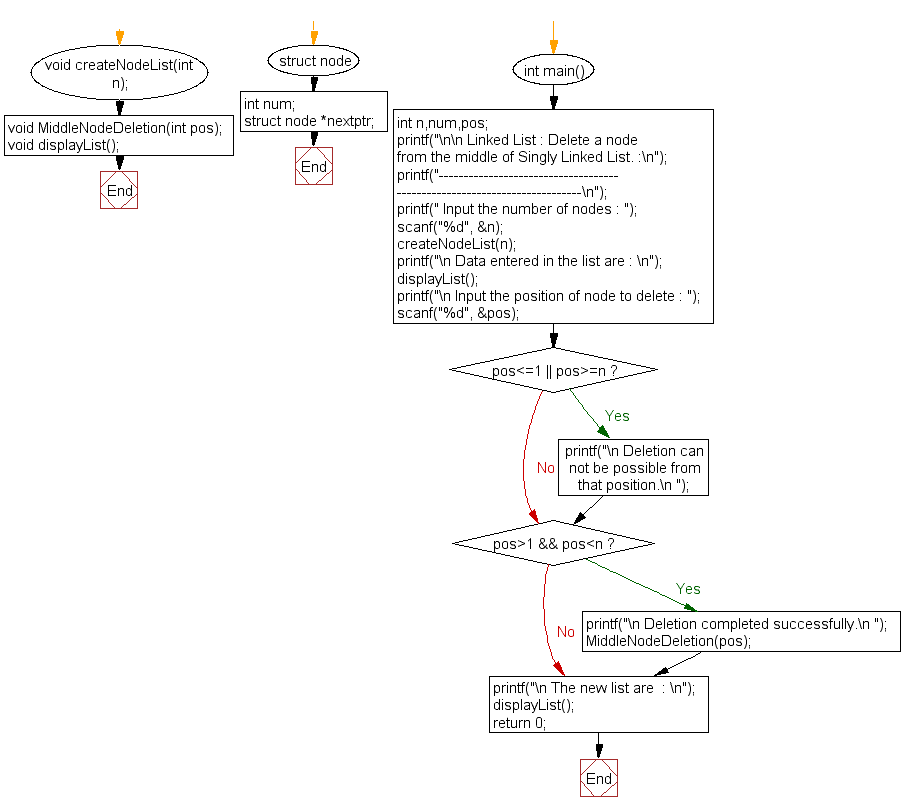createNodeList() :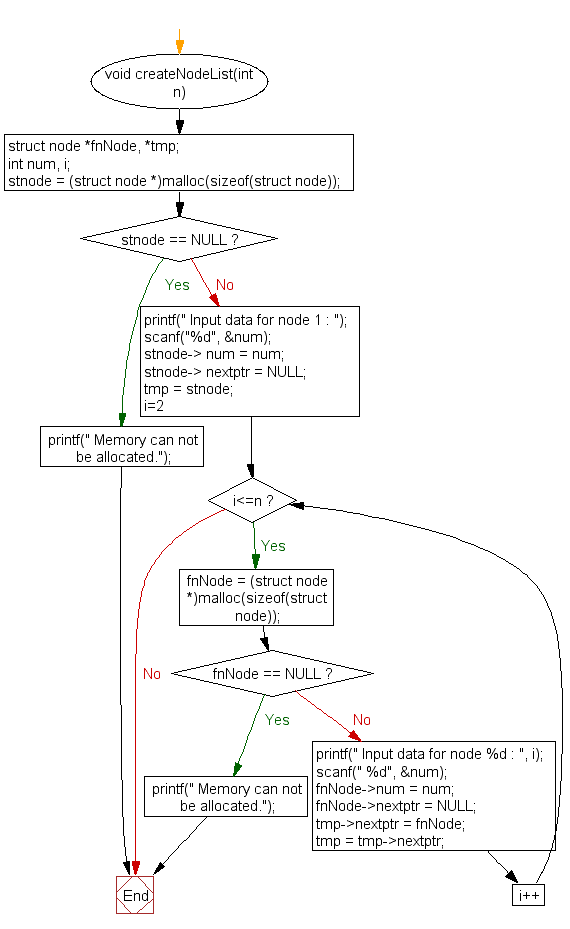MiddleNodeDeletion() :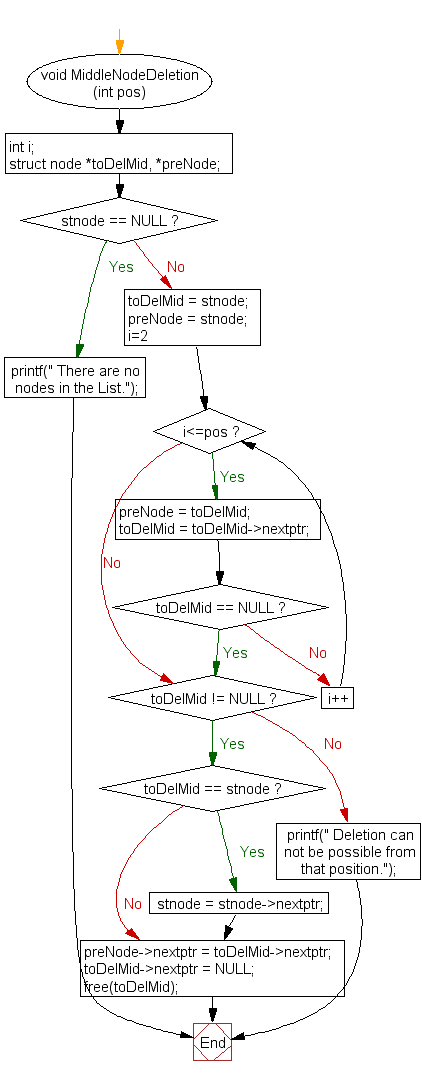displayList() :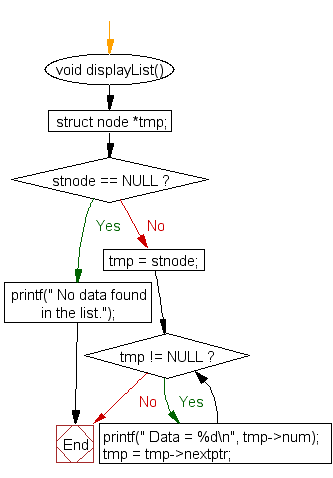C Programming Code Editor:

What is the difficulty level of this exercise?

Test your Programming skills with w3resource's quiz.

﻿